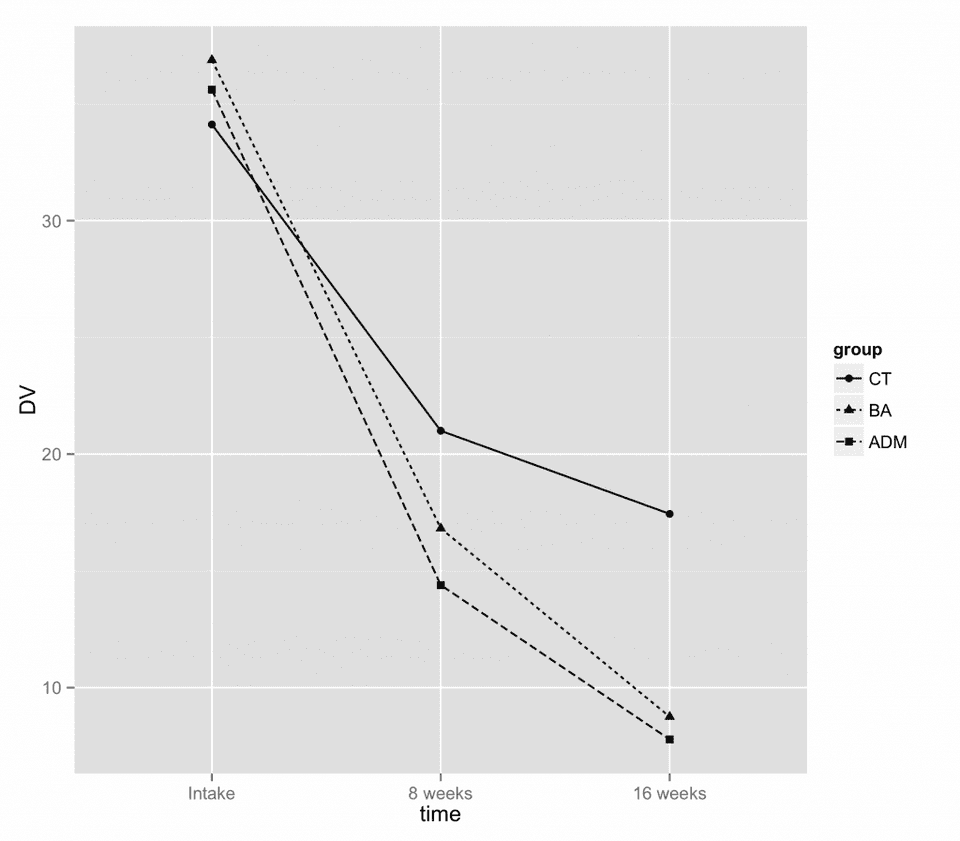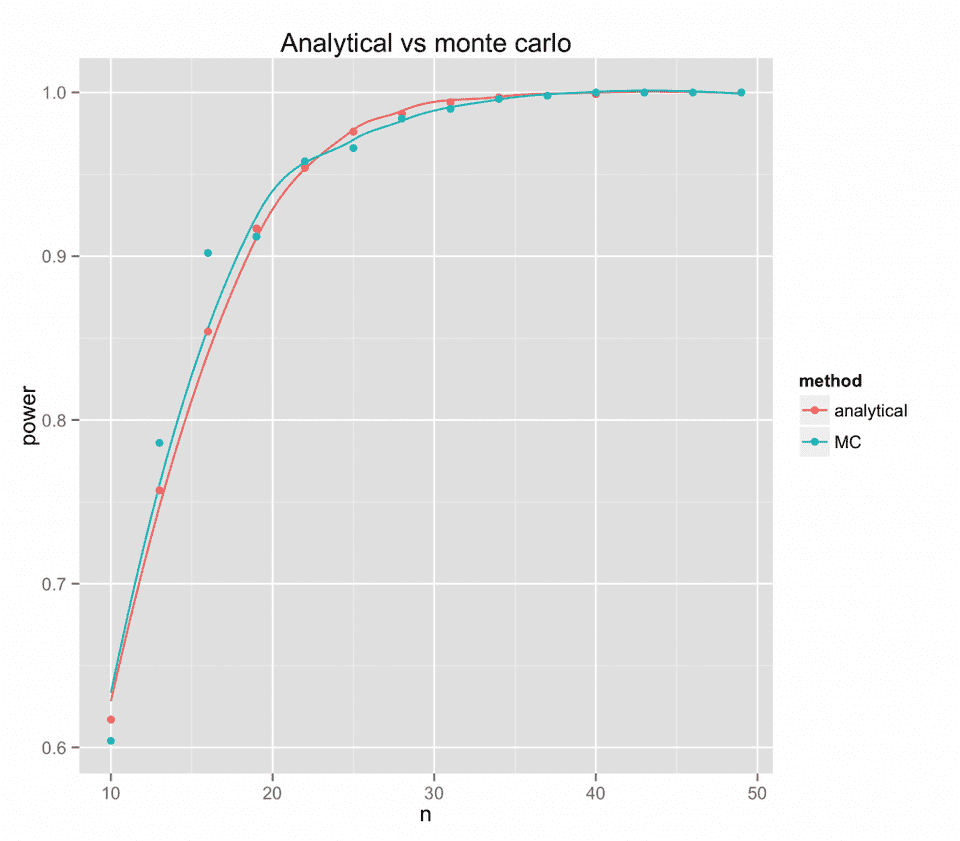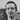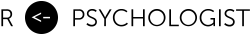# Analytical and simulation-based power analyses for mixed-design ANOVAs

May 22, 2013

In this post I show some R-examples on how to perform power analyses for mixed-design ANOVAs. The first example is analytical — adapted from formulas used in G*Power (Faul et al., 2007), and the second example is a Monte Carlo simulation. The source code is embedded at the end of this post.

Both functions require a dataframe, containing the parameters that will be used in the power calculations. Here is an example using three groups and three time-points.

Here is a plot of our hypothetical study design.Now, we will use this design to perform a power analysis using anova.pwr.mixed and anova.pwr.mixed.sim.

## Comparison of analytical and monte carlo power analysis

Now let’s compare the two functions’ results on the time x group-interaction. Hopefully, the two methods will yield the same result.Luckily, the analytical results are consistent with the Monte Carlo results.

Faul, F., Erdfelder, E., Lang, A. G., & Buchner, A. (2007). G* Power 3: A flexible statistical power analysis program for the social, behavioral, and biomedical sciences.Behavior research methods, 39(2), 175-191.

## Source code

You can find the source here and here.Written by Kristoffer Magnusson a researcher in clinical psychology. You should follow him on Twitter and come hang out on the friendliest open science discord Git Gud Science.

Share:

Published May 22, 2013 (View on GitHub)